# Understanding Non-Linear Regression

Knowing how to fit the model when you have a curvy data set…

All models are wrong, but some are useful… George Edward Pelham

The goal of regression is to build a model to accurately predict unknown cases.

Regression is usually the process of predicting a continuous variable such as housing prices, salaries of workers, rainfall intensity E.t.c, using historical data.

Basically, there are just two types of regression, see link from IBM:-

. Simple Regression

. Multiple Regression

Both simple and multiple regression could be linear or non-linear.

The linearity of regression is based on the nature of the relationship between independent and dependent variables.

This article assumes the reader has intermediate knowledge of the concepts of simple and multiple regression. But fret not, for a refresher, check out my previous in-depth articles on simple and multiple linear regression.

We all love the sight of a scatter plot of our independent and dependent variables that shows an almost distinct straight line to fit our model, but the truth is in reality, a lot of data sets display varying patterns.

So, if the data set shows a curvy trend, then indeed a linear regression model may be unsuitable. In such situations, we need to employ a non-linear regression model. We shall see a few examples in a minute…

## Non-Linear Regression (NLR):

NLR is any relationship between an independent variable X and a dependent variable y which results in a non-linear function modelled data.

Essentially any relationship that is not linear, can be termed as non-linear and is usually represented by the polynomial of k degrees (maximum power of X).

In fact, many different NLRs exist that may be used to fit whatever the data set looks like and these can go on and on to infinite degrees.

Collectively, we can safely call all of these NLRs, polynomial regression, as long as the relationship between the independent variable X and the dependent variable y is modelled as an nth degree polynomial in X. See link from IBM

## So What is Polynomial Regression or Non-Linear Regression?

Polynomial regression fits a curve line to your data. A simple example of a polynomial with a degree of 3 can be shown as:-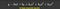where b0 is the intercept or bias unit and b1 to b3 are the slopes of each independent value of variable x.

It sure looks like a feature set for a multiple linear regression right? Just like the one below, Yes, it does. Indeed a polynomial regression is a special case of multiple linear regression, with the main idea of ‘how do you select your features?’.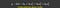where b0 is the intercept or bias unit and b1 to b3 are the slopes of each independent variable x1 to x3

## Common Types of Non-Linear Regression:

Before we go on, let’s briefly look at linear regression. It is of the equation:-

y = b0 + b1x1

Linear regression models a relationship between a dependent variable y and the independent variable x. This relationship has a degree of 1.

As earlier mentioned, There are many types of non-linear regression, but perhaps the most common are:-

. Cubic

. Exponential

. Logarithmic

. Sigmoidal / Logistic

Let’s briefly look at these…

## 1. Cubic:

A cubic function is of the form:- y_hat is equal to intercept plus variable x raised to the third power plus x raised to the second power and so on. It could also be in reverse from 1st power to 3rd power

The graph of this function is not a straight line over the 2D plane. Let’s plot one, but first, take a look at the cubic equation below.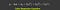y_hat = intercept + x raised to power 3 + x raised to power 2 + x …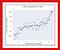Sample Cubic Regression Chart

A quadratic function is of the equation:- y_hat is equal to variable x multiplied by variable x or raised to the power of 2.

## 3. Exponential:

An exponential function with base c is defined as y-hat is equal to intercept plus slope multiplied by a constant(c) which is raised to the power of variable X. See expression below.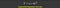where b != 0, c > 0 != 1, x is a variable and a real number and c is also a constant.

Exponential might seem a bit confusing, but plotting it is pretty straight forward… Simply apply the numpy.exp() function and pass variable X as its argument in this form:- y_hat = np.exp(X). Then plot variable X on the x-axis and variable y on the y-axis.

## 4. Logarithmic:

In logarithmic function, y_hat is a result of applying a logarithmic map on variable X. It is one of the simplest expressions of a logarithmic function.

## 5. Sigmoidal / Logistic:

Logistic regression is a variation of linear regression, useful when the observed dependent variable y, is a categorical variable. It fits a special S-shaped curve by taking the linear regression and transforming the numeric estimates into a probability score, using the sigmoid function. See link

With many types of regressions to choose from, there is a good chance that one will fit your data set well.

Remember, it is important to pick a regression model that fits the data set the best.

I’m sure you have a few questions and I would generously answer what I think is the most obvious question…

## Question:

How can I know if a problem is linear or non-linear in an easy way?

To answer the above question, we could do two things.

A.

Visually figure out if the relationship is linear or non-linear. It’s best to plot bivariate plots of output variables with each input variable. See link on bivariate plots on Kaggle

B.

Another easy option is to calculate the correlation coefficient between independent and dependent variables. This could easily be done in pandas by calling the .corr() function on the data set. If for all variables the coefficient is 0.7 or higher, there is a linear tendency and thus a non-linear model is inappropriate.

Okay, Enough Said!! Let’s get our hands dirty with some real live data…

We shall attempt to fit a non-linear model to data points corresponding to China’s GDP from 1960 to 2014. Our data set contains two columns, the first contains the years from 1960 to 2014, the second contains the corresponding Gross Domestic Product (GDP) values for each year.

This is a small data set with 55 rows and 2 columns, but it will suffice.

See link to the data set here in Github.

`# Import librariesimport numpy as npimport pandas as pdimport matplotlib.pyplot as pltchina_gdp = '  https://raw.githubusercontent.com/Blackman9t/Machine_Learning/master/china_gdp.csv'df = pd.read_csv(china_gdp)df.head(10)`

Next, we need to plot a bivariate graph of the data points. The independent variable X (Year) on the x-axis, and the dependent variable y (Value) on the y-axis.

`plt.figure(figsize=(8,5))X_data, y_data = (df['Year'].values, df['Value'].values)plt.plot(X_data, y_data, 'ro')plt.suptitle('Graph showing corresponding years and GDP values for China', y=1.02)plt.ylabel('GDP')plt.xlabel('Year')plt.show()`

Hmmm… This looks kind of familiar. Can you guess which of the NLR charts we explored earlier has a similar curve as the data points above?

If you said Exponential or Logistic… you’re wrong… I’m kidding! of course, you’re right!

It sure looks like Exponential or Logistic… The GDP growth starts off slow and then from 2005 onward, the growth is very significant, and then it decelerates slightly in the 2010s.

## Choosing a model:

The Logistic function could be a good approximation since it has the property of starting slow, increasing growth in the middle and then decreasing again at the end.

## Building the model:

From the sigmoid equation defined above, remember that Beta_1 controls the steepness of the curve, while Beta_2 slides the curve on the x-axis.

Now let’s build our regression model and initialise its’ parameters.

`def sigmoid(X, Beta_1, Beta_2):    """ This method performs the sigmoid function on param X and     Returns the outcome as a varible called y"""    y = 1 / (1 + np.exp(-Beta_1*(X-Beta_2)))    return y`

Let’s now test our sigmoid function with some sample values

`beta_1 = 0.10beta_2 = 1990.0# logistic_functiony_pred = sigmoid(X_data, beta_1, beta_2)# Plot initial predictions against data points.plt.figure(figsize=(8,5))plt.suptitle('Sample Plot: Sigmoid Function on data points')plt.plot(X_data, y_pred*15000000000000.0)plt.plot(X_data, y_data, 'ro')plt.show()`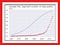The blue line is our sample sigmoid model the red dots are the data points.

## Normalizing our variables:

At this point, let’s normalize our variables

`xdata = X_data / max(X_data)ydata = y_data / max(y_data)`

## Finding the best parameters:

Our next task is to find the best parameters for the non-linear or logistic model. We shall use the curve_fit() method from scipy library. What this method does is:- It uses non-linear least squares estimate to fit the sigmoid function we defined above to the data points.

`from scipy.optimize import curve_fitpopt, pcov = curve_fit(sigmoid, xdata, ydata)# popt are our new optimized parameters# pcov represents the covarianceprint('beta_1 = %f, beta_2 = %f' % (popt,popt))>>  beta_1 = 690.453017, beta_2 = 0.997207`

So now that we have the ideal parameters, thanks to the curve_fit() method, we shall use them to fit our model, in other to minimize the sum of squared differences between each prediction and its corresponding actual value.

`x = np.linspace(1960, 2015, 55)# Normalize xx = x / max(x)plt.figure(figsize=(8,5))y = sigmoid(x, popt, popt)# Plotting the original data pointsplt.plot(xdata, ydata, 'ro', label='data')# Plotting the fitted prediction lineplt.plot(x, y, linewidth=3.0, label='fit')plt.legend(loc='best')plt.ylabel('GDP', color='r', fontsize=18)plt.xlabel('Year', color='r', fontsize=18)plt.xticks(color = 'y')plt.yticks(color = 'y')plt.show()`

As we can see it looks like a pretty good fit, but let’s evaluate our model…

First, let’s split the data into a training and testing data set.

`msk = np.random.rand(len(df)) < 0.8 train_x = xdata[msk] test_x = xdata[~msk] train_y = ydata[msk]test_y = ydata[~msk]`

Next, we build the model using the training set to extract ideal params

`popt, pcov = curve_fit(sigmoid, train_x, train_y)# Remember popt saves the ideal parameters from curve_fit method# While pcov stores the covarianceprint('Ideal params are: ', popt)>>Ideal params are:  [670.91888462   0.99708276]`

Now, we make the predictions using the test set

`y_hat = sigmoid(test_x, *popt)# *popt means unpack popt into popt and popt`

## Evaluation…

`mean_abs_error = np.mean(np.absolute(y_hat - test_y))mean_squ_error = np.mean(np.absolute((y_hat - test_y) **2))print("Mean absolute error: %.2f" % mean_abs_error)print("Residual sum of squares (MSE): %.2f" % mean_squ_error)# Next let's check the R2 score, The coefficient of determinationfrom sklearn.metrics import r2_scorer_score = r2_score(y_hat, test_y)print("R2-score: %.2f" % r_score)>>Mean absolute error: 0.04 Residual sum of squares (MSE): 0.00 R2-score: 0.95`

MAE = 0.4; MSE = 0.0. ; R2-score = 0.95 (95%)

## Summary:

It takes a good dose of some practice, but clearly, as we’ve seen with this small data set, it is actually possible to fit a non-linear regression line through a curvy data set. Python has an abundance of modules to help us fit a model to predict a continuous or even a categorical variable.

Feel free to go through the notebook on Github for more details, especially on plotting the NLR charts we did earlier.

Cheers!

Written by

## Towards AI

#### Towards AI, is the world’s fastest-growing AI community for learning, programming, building and implementing AI.

Welcome to a place where words matter. On Medium, smart voices and original ideas take center stage - with no ads in sight. Watch
Follow all the topics you care about, and we’ll deliver the best stories for you to your homepage and inbox. Explore
Get unlimited access to the best stories on Medium — and support writers while you’re at it. Just \$5/month. Upgrade